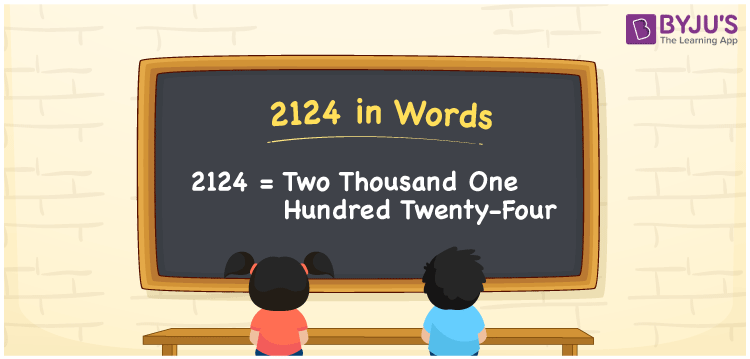# 2124 in Words

2124 in words is expressed as Two Thousand One Hundred Twenty-Four. If you bought a dress material worth Rs. 2124, you can say, “I bought a dress material worth Rupees Two Thousand Two Hundred Twenty-Four”. 2124 in words can be written using the English alphabet. The number 2124 is a cardinal number since it denotes an exact quantity. Let us learn how to write and spell the number 2124 in English in this article.

 2124 in Words Two Thousand One Hundred Twenty-Four Two Thousand One Hundred Twenty-Four in numerical form 2124

## 2124 in English Words

Generally, the English alphabet is used to write numbers in words. Hence, we can read the number 2124 in English as Two Thousand One Hundred Twenty-Four.## How to Write 2124 in Words?

Since there are four digits in the number 2124. So, let us create a chart of four columns. The place value chart for the number 2124 is as follows:

 Thousands Hundreds Tens Ones 2 1 2 4

Therefore, we can write the expanded form as:

2 x Thousand + 1 x Hundred + 2 x Ten + 4 x One

= 2 x 1000 + 1 x 100 + 2 x 10 + 4 x 1

= 2000 + 100 + 20 + 4

= 2124

= Two Thousand One Hundred Twenty-Four

Thus, 2124 in words is written as Two Thousand One Hundred Twenty-Four.

Interesting way of writing 2124 in words

2 = Two

21 = Twenty-One

212 = Two Hundred and Twelve

2124 = Two Thousand One Hundred Twenty-Four

Hence, the word form of the number 2124 is Two Thousand One Hundred Twenty-Four.

2124 is a natural number that precedes 2125 and succeeds 2123

• 2124 in words – Two Thousand One Hundred Twenty-Four
• Is 2124 an odd number? – No
• Is 2124 an even number? – Yes
• Is 2124 a perfect square number? – No
• Is 2124 a perfect cube number? – No
• Is 2124 a prime number? – No
• Is 2124 a composite number? – Yes

## Frequently Asked Questions on 2124 in Words

Q1

### Write 2124 in words.

2124 in words is written as Two Thousand One Hundred Twenty-Four.
Q2

### What is the value of 1000 plus 1124?

1000 + 1124 = 2124 Therefore, the value of 1000 plus 1124 is 2124.
Q3

### 2124 is a composite number. True or False.

True, 2124 is a composite number.